# Jacobian

In mathematics, the Jacobi matrix is the matrix of first-order partial derivatives of the (vector-valued) function: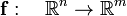$\mathbf{f}:\quad\mathbb{R}^n \rightarrow \mathbb{R}^m$

(often f maps only from and to appropriate subsets of these spaces). The Jacobi matrix is m × n, i.e., consists of m rows and n columns. Row k contains the first-order partial derivatives of fk with respect to x1, ...,xn, respectively. The Jacobi matrix is also known as the functional matrix of Jacobi. The determinant of the Jacobi matrix for n = m is known as the Jacobian. The Jacobi matrix and its determinant have several uses in mathematics:

• For m = 1 (f is a scalar valued function of n variables), the Jacobi matrix appears in the second (linear) term of the Taylor series of f. Here the Jacobi matrix is 1 × n (the gradient of f, a row vector).
• The inverse function theorem states that if m = n and f is continuously differentiable, then f is invertible in the neighborhood of a point x0 if and only if the Jacobian at x0 is non-zero.

The Jacobi matrix and its determinant are named after the German mathematician Carl Gustav Jacob Jacobi (1804 - 1851).

## Definition

Let f be a map of an open subset T of$\mathbb{R}^n$ into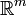$\mathbb{R}^m$ with continuous first partial derivatives,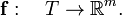$\mathbf{f}:\quad T \rightarrow \mathbb{R}^m.$

That is if$\mathbf{t} = (t_1,\; t_2,\; \ldots, t_n)\in T \sub \mathbb{R}^n,$

then\begin{align} x_1 &= f_1(t_1, t_2,\ldots, t_n) \\ x_2 &= f_2(t_1, t_2,\ldots, t_n) \\ \cdots & \cdots\\ x_m &= f_m(t_1, t_2,\ldots, t_n), \\ \end{align}

with$\mathbf{x} = (x_1,\; x_2,\; \ldots, x_m)\in \mathbb{R}^m .$

The m × n functional matrix of Jacobi consists of partial derivatives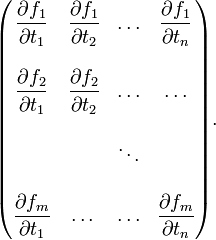$\begin{pmatrix} \dfrac{\partial f_1}{\partial t_1} & \dfrac{\partial f_1}{\partial t_2} & \ldots &\dfrac{\partial f_1}{\partial t_n} \\ \\ \dfrac{\partial f_2}{\partial t_1} & \dfrac{\partial f_2}{\partial t_2} & \ldots &\dots\\ \\ & &\ddots\\ \\ \dfrac{\partial f_m}{\partial t_1} & \dots & \ldots &\dfrac{\partial f_m}{\partial t_n}\\ \end{pmatrix} .$

The determinant (which is only defined for square matrices) of this matrix is usually written as (take m = n),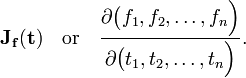$\mathbf{J}_\mathbf{f}(\mathbf{t})\quad\hbox{or}\quad \frac{\partial\big(f_1, f_2,\ldots, f_n \Big)}{\partial \big(t_1,t_2,\ldots, t_n\Big)} .$

### Example

Let T be the subset {r, θ, φ | r > 0, 0 < θ<π, 0 <φ <2π} in ℝ3 and let f be defined by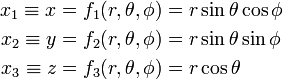\begin{align} x_1 \equiv x &= f_1(r,\theta, \phi) = r\sin\theta\cos\phi \\ x_2 \equiv y &= f_2(r,\theta, \phi) = r\sin\theta\sin\phi \\ x_3 \equiv z &= f_3(r,\theta, \phi) = r\cos\theta \\ \end{align}

The Jacobi matrix is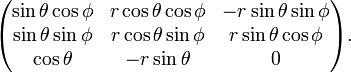$\begin{pmatrix} \sin\theta\cos\phi & r\cos\theta\cos\phi & -r\sin\theta\sin\phi \\ \sin\theta\sin\phi & r\cos\theta\sin\phi & r\sin\theta\cos\phi \\ \cos\theta & -r\sin\theta & 0 \\ \end{pmatrix} .$

Its determinant can be obtained most conveniently by a Laplace expansion along the third row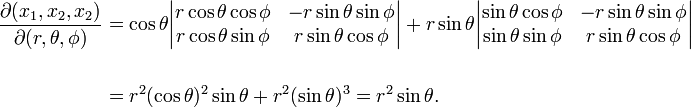\begin{align} \frac{\partial(x_1, x_2, x_2)}{\partial(r, \theta, \phi)} &= \cos\theta \begin{vmatrix} r\cos\theta\cos\phi & -r\sin\theta\sin\phi \\ r\cos\theta\sin\phi &r\sin\theta\cos\phi \end{vmatrix} +r\sin\theta \begin{vmatrix} \sin\theta\cos\phi & -r\sin\theta\sin\phi \\ \sin\theta\sin\phi &r\sin\theta\cos\phi \end{vmatrix} \\ \\ & = r^2(\cos\theta)^2 \sin\theta + r^2 (\sin\theta)^3 = r^2\sin\theta . \end{align}

The quantities {r, θ, φ} are known as spherical polar coordinates and its Jacobian is r2sinθ.

## Coordinate transformation

Let$T \sub \mathbb{R}^n$. The map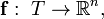$\mathbf{f}:\; T \rightarrow \mathbb{R}^n,$ is a coordinate transformation if (i) f has continuous first derivatives on T (ii) f is one-to-one on T and (iii) the Jacobian of f is not equal to zero on T.

## Multiple integration

It can be proved  that$\int_{\mathbf{f}(\mathbf{t})} \phi(\mathbf{x})\; \mathrm{d}\mathbf{x} =\int_T \phi\big(\mathbf{f}(\mathbf{t})\big)\; \mathbf{J}_\mathbf{f}(\mathbf{t})\;\mathrm{d}\mathbf{t}.$

As an example we consider the spherical polar coordinates mentioned above. Here x = f(t) ≡ f(r, θ, φ) covers all of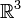$\mathbb{R}^3$, while T is the region {r > 0, 0 < θ<π, 0 <φ <2π}. Hence the theorem states that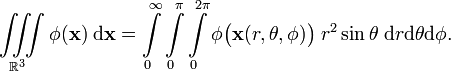$\iiint\limits_{\mathbb{R}^3} \phi(\mathbf{x})\; \mathrm{d}\mathbf{x} = \int\limits_{0}^\infty \int\limits_0^\pi \int\limits_0^{2\pi} \phi\big(\mathbf{x}(r,\theta,\phi)\big)\; r^2\sin\theta \; \mathrm{d}r \mathrm{d}\theta \mathrm{d}\phi .$

## Geometric interpretation of the Jacobian

The Jacobian has a geometric interpretation which is illustrated for the example n = 3.

The following is a vector of infinitesimal length in the direction of increase in t1,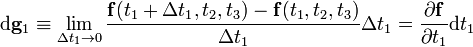$\mathrm{d}\mathbf{g}_1 \equiv \lim_{\Delta t_1 \rightarrow 0} \frac{\mathbf{f}(t_1+\Delta t_1, t_2, t_3) - \mathbf{f}(t_1, t_2, t_3)}{\Delta t_1}\Delta t_1 = \frac{\partial \mathbf{f}}{\partial t_1} \mathrm{d}t_1$

Similarly, we define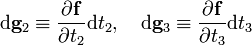$\mathrm{d}\mathbf{g}_2 \equiv \frac{\partial \mathbf{f}}{\partial t_2} \mathrm{d}t_2,\quad \mathrm{d}\mathbf{g}_3 \equiv \frac{\partial \mathbf{f}}{\partial t_3} \mathrm{d}t_3$

The scalar triple product of these three vectors gives the volume of an infinitesimally small parallelepiped,$\mathrm{d}V = \mathrm{d}\mathbf{g}_1 \cdot ( \mathrm{d}\mathbf{g}_2\times \mathrm{d}\mathbf{g}_3 ) = \frac{\partial \mathbf{f}}{\partial t_1} \cdot \left(\frac{\partial \mathbf{f}}{\partial t_2} \times \frac{\partial \mathbf{f}}{\partial t_3}\right) \; \mathrm{d}t_1\mathrm{d}t_2\mathrm{d}t_3$

The components of the first vector are given by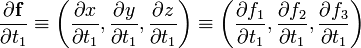$\frac{\partial \mathbf{f}}{\partial t_1} \equiv \left( \frac{\partial x}{\partial t_1}, \frac{\partial y}{\partial t_1}, \frac{\partial z}{\partial t_1} \right) \equiv \left( \frac{\partial f_1}{\partial t_1}, \frac{\partial f_2}{\partial t_1}, \frac{\partial f_3}{\partial t_1} \right)$

and similar expressions hold for the components of the other two derivatives. It has been shown in the article on the scalar triple product that$\frac{\partial \mathbf{f}}{\partial t_1} \cdot \left(\frac{\partial \mathbf{f}}{\partial t_2} \times \frac{\partial \mathbf{f}}{\partial t_3}\right) = \begin{vmatrix} \dfrac{\partial f_1}{\partial t_1} & \dfrac{\partial f_2}{\partial t_1} & \dfrac{\partial f_3}{\partial t_1} \\ \dfrac{\partial f_1}{\partial t_2} & \dfrac{\partial f_2}{\partial t_2} & \dfrac{\partial f_3}{\partial t_2} \\ \dfrac{\partial f_1}{\partial t_3} & \dfrac{\partial f_2}{\partial t_3} & \dfrac{\partial f_3}{\partial t_3} \\ \end{vmatrix} \equiv \frac{\partial( f_1, f_2, f_3)}{\partial( t_1, t_2, t_3)} \equiv \mathbf{J}_{\mathbf{f}}(\mathbf{t}).$

Note that a determinant is invariant under transposition (interchange of rows and columns), so that the transposed determinant being given is of no concern. Finally.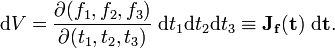$\mathrm{d}V = \frac{\partial( f_1, f_2, f_3)}{\partial( t_1, t_2, t_3)}\; \mathrm{d}t_1\mathrm{d}t_2\mathrm{d}t_3 \equiv \mathbf{J}_{\mathbf{f}}(\mathbf{t})\; \mathrm{d}\mathbf{t} .$

## Reference

1. T. M. Apostol, Mathematical Analysis, Addison-Wesley, 2nd ed. (1974), sec. 15.10